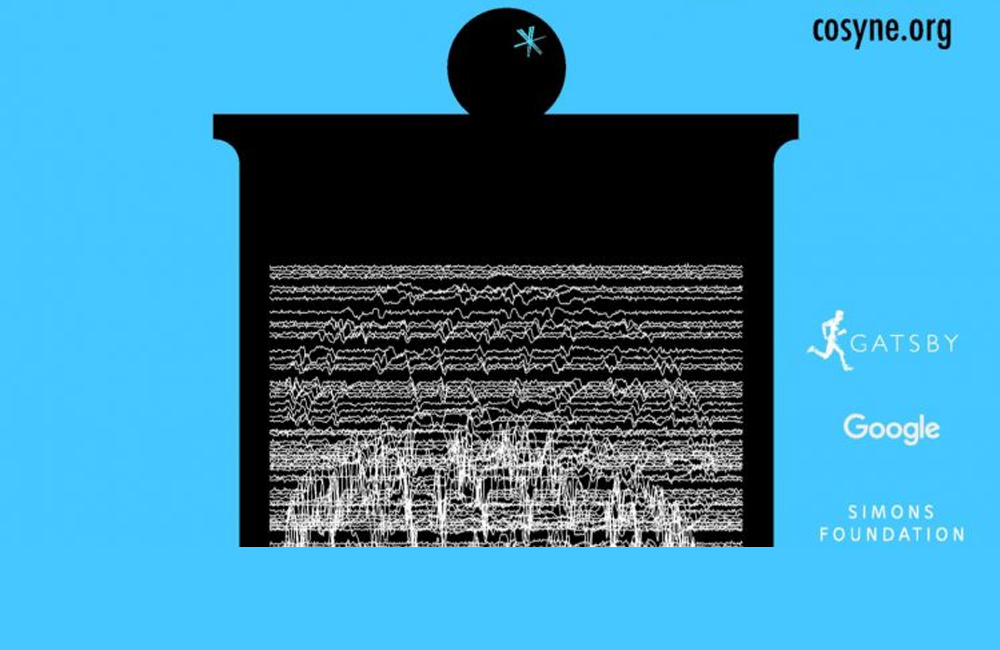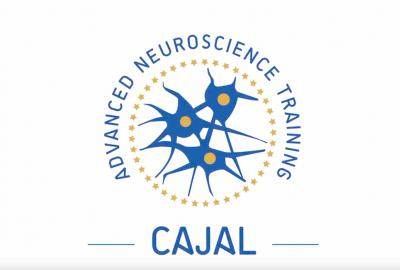Statistical models

By
Category
Level
Beginner

Introductory lectures on different aspects of statistical models.

Introduction to the neural coding problem
Aspects of neural activity carry information
Multiple spike train models
Regularization
Beyond GLM
Latent variable model
Lessons of this Course
1
Duration:
1:45:48

Part 1 of 2 of a tutorial on statistical models for neural data

2
Duration:
1:50:31

Part 2 of 2 of a tutorial on statistical models for neural data.

Recent coursesData science and reproducibilityCajal Course in Computational Neuroscience